Get instant live expert help with Excel or Google Sheets“My Excelchat expert helped me in less than 20 minutes, saving me what would have been 5 hours of work!”

#### Post your problem and you’ll get Expert help in seconds.

Your message must be at least 40 characters
Our professional Expert are available now. Your privacy is guaranteed.

# Finding data range in Excel

A data range in Excel is simply a collection of two or more cells; the cells are not required to be next to each other.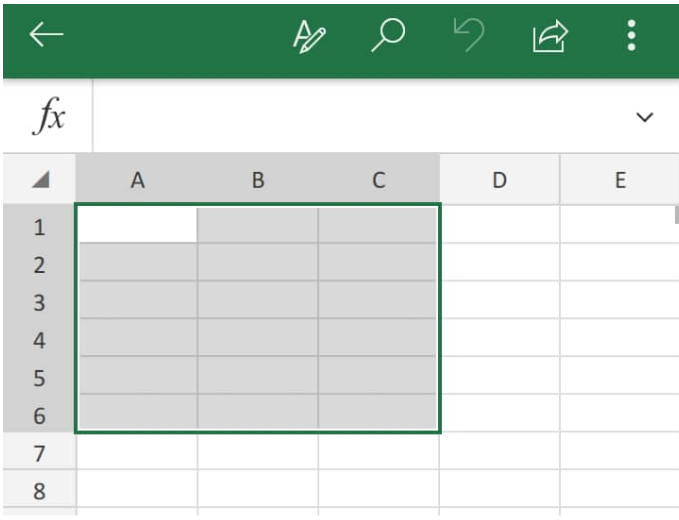Figure 1. Cell Range in Excel

## Generic Formula

`=RANGE(max value-min value)`

• Max value = Largest number value in the selected range
• Min value = Smallest number value in the selected range

We can select a cell range in Excel by holding down the CTRL keyboard key and then clicking on each individual cell that we want to be included in the range. The data range in Excel of a particular set of data gives us a simple idea of the distribution of values that it contains.

## How to Calculate Range in Excel

Finding Range in Excel is a simple task which requires us to subtract the Minimum value of numbers contained in the selected cells from the Maximum value.

Here’s how to calculate range in Excel;

1. We begin by finding the MAX value of a set of data in our worksheet;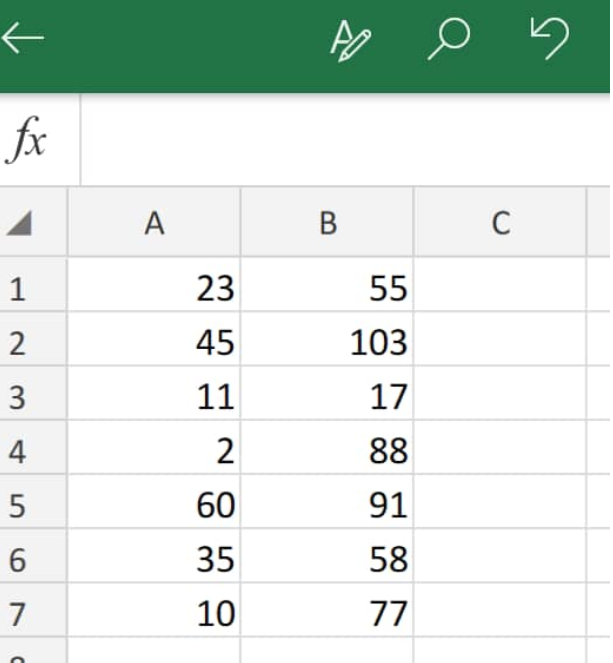Figure 2. Data Values in Excel

To determine the MAX value for the data set above we will use the MAX Function in Excel.

1. The MAX formula we will enter into cell B9 of our worksheet example is as follows;
`=MAX(A1:B7)`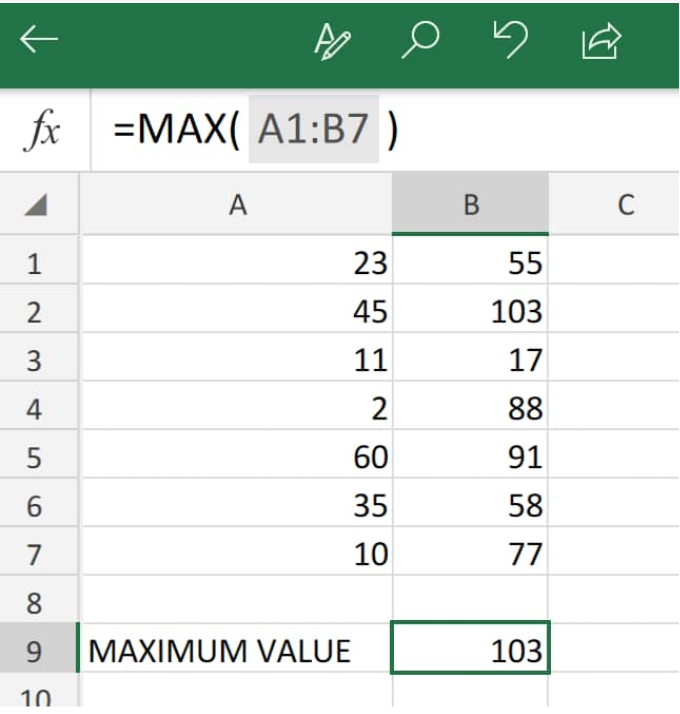Figure 3. MAX Value in Excel

The range function in Excel returned the MAX value in the selected  range of data above (A1:B7), as103.

1. Next, we have to determine the MIN value in our data set.
The MIN formula we will enter into cell B9 of our worksheet example is as follows;
`=MIN(A1:B7)`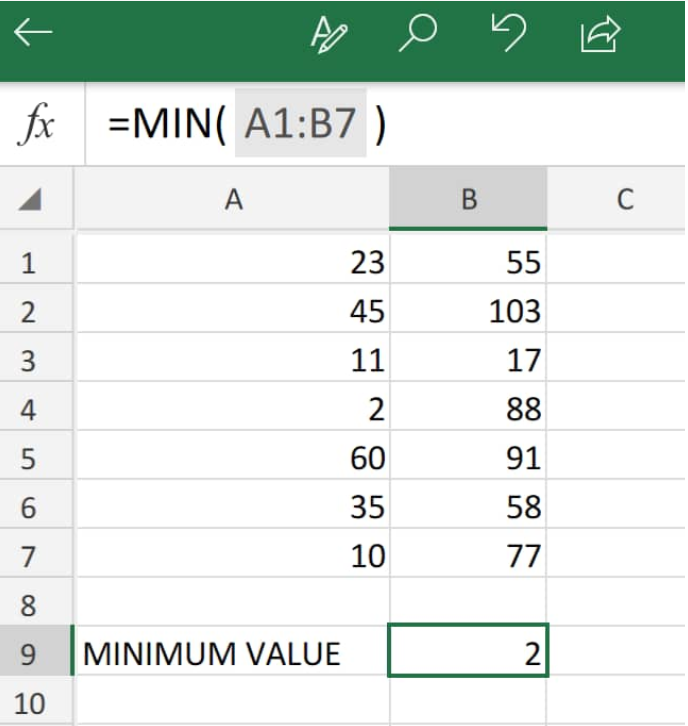Figure 4. MIN Value in Excel

The range function in Excel returned the MIN value in the selected  range of data above (A1:B7), as 2.

Since we now know how to use the range function in Excel to calculate MAX and MIN values, let us now demonstrate how to find the range in Excel by subtracting the MIN value from the MAX value in the following data set;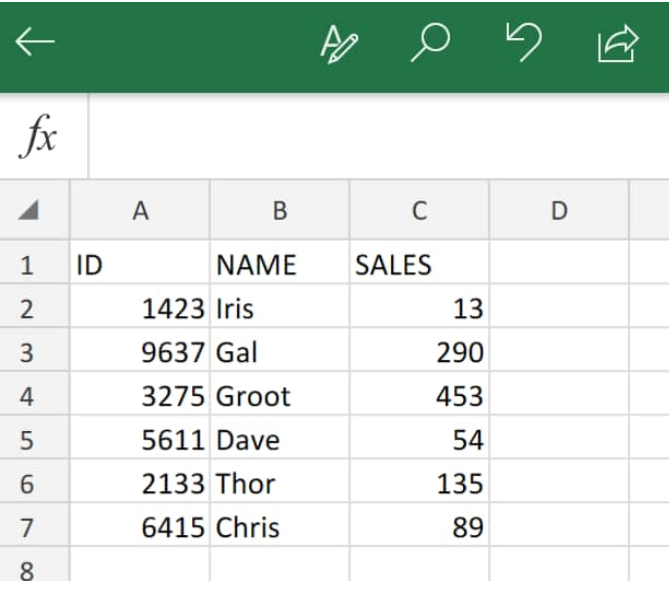Figure 5. Data Set in Excel

1. The Excel range formula we will enter into cell B9 of our worksheet example is as follows;
`=MAX(C2:C7)-MIN(C2-C7)`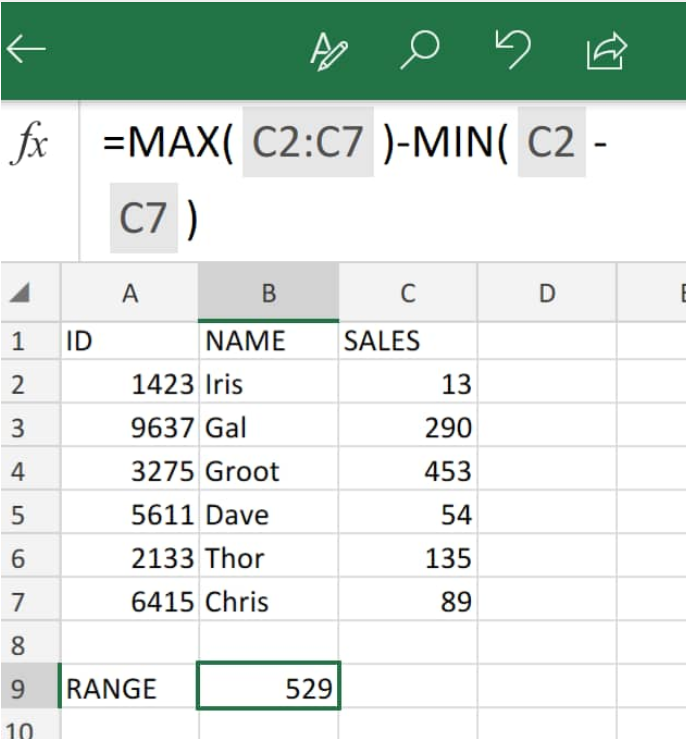Figure 6. Finding Range in Excel

The Excel range function returned 529 as the total range value after subtracting the MIN value from the MAX value in the data set above.

## Instant Connection to an Excel Expert

Most of the time, the problem you will need to solve will be more complex than a simple application of a formula or function. If you want to save hours of research and frustration, try our live Excelchat service! Our Excel Experts are available 24/7 to answer any Excel question you may have. We guarantee a connection within 30 seconds and a customized solution within 20 minutes.